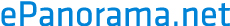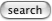# Coils

## Coil equations

### General formula

Equation for calculating inductance of a homemade coil: L=(D*N^2)/(l/D+0,43)

Where

• D is diameter in cm
• l i length in cm
• L is inductance in uH
• N is nuber of turns

### Air core coils

L = (r^2 * n^2)/(9r + 10l)

For air core coils you can come close with:

• L = ind. in uH
• r = radius of coil in inches
• n = number of turns
• l = coil length in inches
ref: Bauchbaum's Complete Handbook of Practical Electronic Ref. Data

Air cores typically range from .1 to 2000 uH. Bigger indictances usually make the coil too bulky and the above formula is not accurate enough because inner and outer radii of your windings may vary too widely.

### Another coil equation

L = (a^2*n^2)/(9*a+10*b)

where

• a = radius in inches
• b = length in inches
• n = number of turns
this was claimed to be accurate within a few percent.

### Equation for single layer coils

L= N^2*A*u*u0/l

Where:

• L=inductance desired
• N=Number of turns
• A=cross sectional area of core in square centimeters
• u(Mu)=permeability of core (Air=1; Iron~1000)
• u0 (mu subzero)= Absolute permeability of air (1.26*10^-12)
• l=length of coil in centimeters

#### Simple formulas for turn numbers of air and iron core coils

Air core: N=(SR[A*u*u0/l])*20Pi

Iron core: N=(SR[A*u*u0/1*.5Pi])

Where:

• N=number of turns needed for coil
• SR=Square Root (of bracketed equation)
• A=cross sectional area of core
• u=Permeability of core
• u0=Absolute permeability of air
• l=length of coil
• 20Pi=20 times Pi or about 63
• .5Pi=half of Pi or 1.57

The equations "sort of" govern the inductance. Testing with inductance meters will ascertain desired inductance.

## Program for calculating coil inductance

Here is a simple basic program for calculating coils in GW Basic.

```100 'program to calculate the number of turns for an inductor.
110 CLS:PI=3.1415926545#
120 K=1473061.855#               'mhos per square inch of area of copper
130 PRINT "                      ÉÍÍÍÍÍÍÍÍÍÍÍÍÍÍÍÍÍÍÍÍÍÍÍÍÍÍÍÍ»"
140 PRINT "                      | Inductor Design Calculator |"
150 PRINT "                      | by David E. Powell, KA4KNG |"
160 PRINT "                      ÈÍÍÍÍÍÍÍÍÍÍÍÍÍÍÍÍÍÍÍÍÍÍÍÍÍÍÍÍ¼":PRINT:PRINT
210 INPUT "Desired inductance in microhenries";L
220 INPUT "Gauge or diameter of wire in inches";DIAMETER
230 INPUT "Diameter of coil form in inches";FORM
240 IF DIAMETER >= 1 THEN DIAMETER = .46 / 1.1229283027#^(DIAMETER +3)
250 PRINT:PRINT "calculating";
260 LAYERS=1                    'Single layer starting out
500 A=DIAMETER*LAYERS+FORM      'Average coil diameter to center of
thickness
505 PRINT ".";
510 GOSUB 2000                  'calculate the number of turns
520 IF PROB =1 THEN LAYERS = LAYERS+1:IF LAYERS < 100 THEN GOTO 500
530 IF B > 1 THEN LAYERS=LAYERS+1:GOTO 500
540 IF N > 9999 THEN PRINT "ERROR - turns count larger than 10000":SYSTEM
550 N=CINT(N):B=N*DIAMETER/LAYERS:LTRY = (.2 * A^2 * N^2)/(3*A+9*B+10*C)
560 W.LENGTH = N*A*PI
570 'calculate the resistance of coil
580 W.AREA = (DIAMETER/2)^2*PI
590 R = 1/(W.AREA*K)*W.LENGTH
800 'show the results
810 PRINT:PRINT
815 PRINT "Overall coil diameter......  ";DIAMETER*LAYERS*2+FORM;"inches"
820 PRINT "Average coil diameter......  ";A;"inches"
830 PRINT "Depth of coil..............  ";LAYERS*DIAMETER;"inches"
840 PRINT "Length of coil.............  ";B;"inches"
850 PRINT "Length of wire (approx)....  ";INT(W.LENGTH/12)"feet,";
855 PRINT INT((W.LENGTH-INT(W.LENGTH))*12);"inches"
860 PRINT "Number of layers...........  ";LAYERS
870 PRINT "Number of turns............  ";N
880 PRINT "Number of turns per layer..  ";N/LAYERS
890 PRINT "Actual inductance..........  ";LTRY;"microhenries"
900 PRINT "Coil DC resistance.........  ";R;"ohms"
1000 INPUT "Again";ZZZ\$
1010 IF ZZZ\$="n" OR ZZZ\$="N" THEN SYSTEM
1020 GOTO 100
2000 'subroutine to calculate the number of turns
2010 MIN.N=1:MAX.N=10000:C=LAYERS * DIAMETER:PROB=0
2060     N=(MAX.N-MIN.N)/2+MIN.N
2070     B=N*DIAMETER/LAYERS     'length of coil
2080     LTRY = (.2 * A^2 * N^2)/(3*A+9*B+10*C)
2090     IF CINT(MAX.N) = CINT(MIN.N) THEN GOTO 3000
2100     IF MIN.N => 9999 THEN PROB=1:GOTO 3000
2110     IF LTRY < L THEN MIN.N=N:GOTO 2060
2120     IF LTRY > L THEN MAX.N=N:GOTO 2060
3000     RETURN

```

```
```